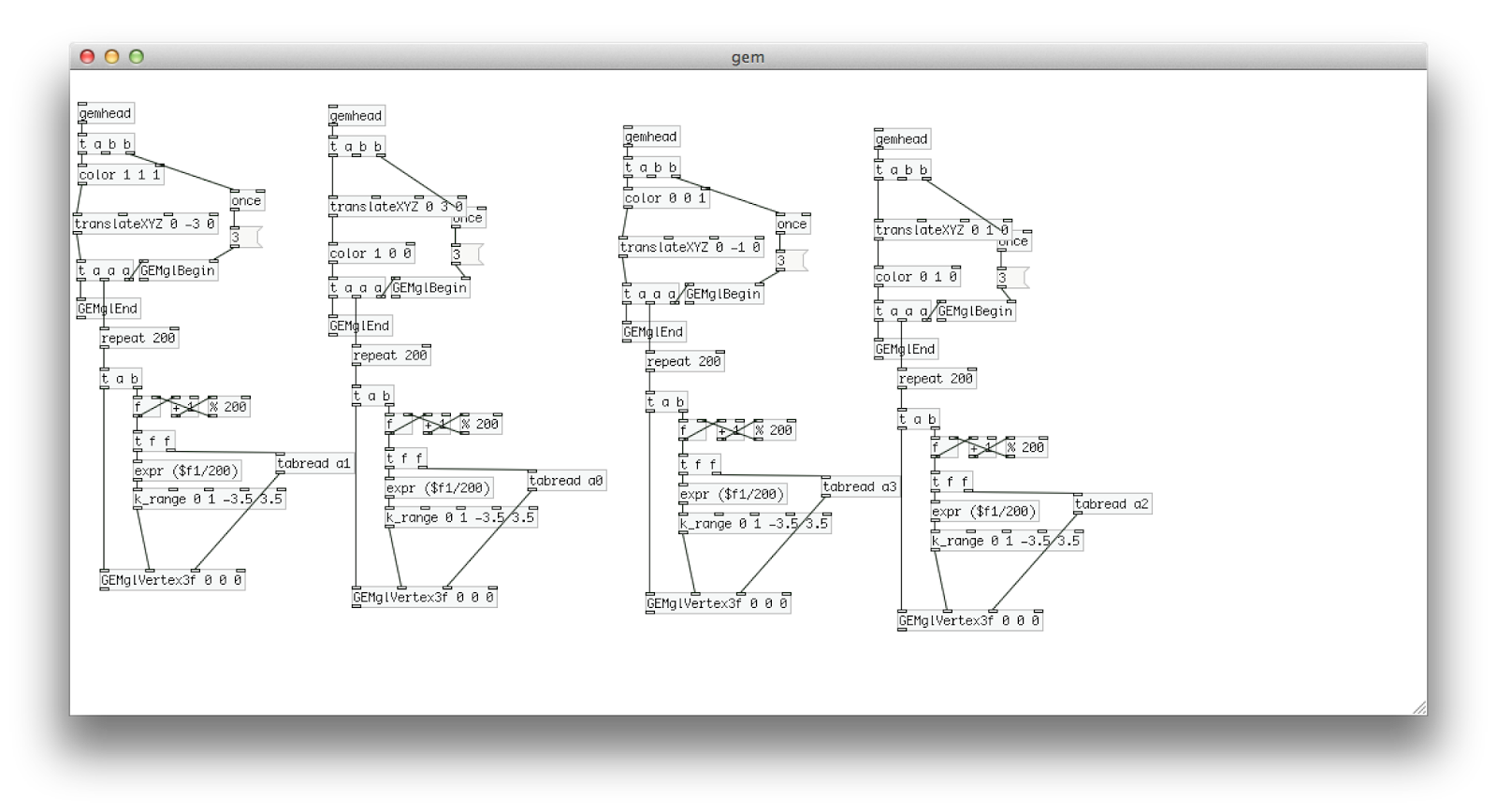## 2014年7月3日 星期四

### [QC] 圓表面運動

延續兩天前的話題，既然連unity都做過圓球表面運動了，那麼很久沒打開都快生鏽發霉的QC（quartz composer）當然也要來一下。QC的優點就是，跟所有的vj軟體配合度都相當高，內部還支援javascript寫作，所以本範例 登"登" ，只花了一個小時就實做出來（對比 unity，我只能嘆聲連連）。
```var first=false;
var x;
var y;
var z;

var theta;
var phi;

var VelT;
var VelP;
var num;

var bx1;
var bx2;
var by1;
var by2;
var bz1;
var bz2;

var mySphere=[];
var myLines=[];

function dis(x1,y1,z1,x2,y2,z2){
return Math.sqrt(Math.pow((x1-x2),2)+Math.pow((y1-y2),2)+Math.pow((z1-z2),2));
}

function (__structure myStructures,__structure myStructures2,__number LinesNum) main (__number time,__number numball,__number limit)
{
var result = new Object();
myLines=[];

if(num != numball){
first=true;
mySphere=[];
num= numball;
}

if(first==true){
for(var i=0;i<num;i++){
theta=(6.28*(Math.random()));
phi=(6.28*(Math.random()));

VelT=0.02*(Math.random())+0.01;
VelP=0.02*(Math.random())+0.01;

x=radius * Math.cos ((theta)) * Math.cos ((phi));
z=radius * Math.sin ((theta)) * Math.cos ((phi));

mySphere.push({ax:x,ay:y,az:z,
atheta:theta,aphi:phi,
avelt:VelT,avelp:VelP
});
first=false;
}
}

for(var i=0;i<mySphere.length;i++){

mySphere[i].atheta = mySphere[i].atheta + mySphere[i].avelt ;
mySphere[i].aphi =    mySphere[i].aphi + mySphere[i].avelp ;
mySphere[i].ax = radius * Math.cos ((mySphere[i].atheta)) * Math.cos ((mySphere[i].aphi));
mySphere[i].ay = radius * Math.sin ((mySphere[i].aphi));
mySphere[i].az = radius * Math.sin ((mySphere[i].atheta)) * Math.cos ((mySphere[i].aphi));  }

for (var i=0; i<mySphere.length; i++) {
for (var j=i+1; j<mySphere.length; j++) {
bx1=mySphere[i].ax;
by1=mySphere[i].ay;
bz1=mySphere[i].az;
bx2=mySphere[j].ax;
by2=mySphere[j].ay;
bz2=mySphere[j].az;

if(dis(bx1,by1,bz1,bx2,by2,bz2)<limit){
myLines.push({lx1:bx1,ly1:by1,lz1:bz1,
lx2:bx2,ly2:by2,lz2:bz2
});

}
}
}

result.myStructures=mySphere;
result.myStructures2=myLines;
result.LinesNum=myLines.length;
return result;
}

```

SoureceCode

## 2014年5月31日 星期六

### http://zhuanlan.zhihu.com/wille/19759362## 2014年4月30日 星期三

### [GLSL]入門-6 把陣列轉換成材質

```uniform sampler2D tex;
uniform vec2 resolution;

vec2 p=uv;

//將索引值換算成座標
p.x=(mod(id,12.0))/ 12.0 + 0.0415;
p.y=1.0-((float(floor(id/12.0))/ 12.0) + 0.0415);

//把座標上的色彩，轉換成灰階值。
float cc= dot(texture2D(tex, p).rgb, vec3(0.2126, 0.7152, 0.0722));

return cc;
}

float rectangle(vec2 uv, vec2 pos, vec2 size) {
float t = 0.0;
if ((uv.x > pos.x - size.x / 2.0) && (uv.x < pos.x + size.x / 2.0)
&& (uv.y > pos.y - size.y / 2.0) && (uv.y < pos.y + size.y / 2.0)) {
t = 0.3;
}
return t;
}

void main(void)
{ vec4 color;
vec2 uv = (gl_FragCoord.xy/resolution.xy);

//讓材質只畫在左下角
if(uv.x<0.25 && uv.y<0.25){
color = texture2D(tex, fract(uv*4.0)).rgba;
}else{
color =vec4(0.0);
}

float rect=0.0;

for(float i=0.0;i<36.0;i++){
rect += rectangle(uv,vec2(a,b),vec2(c,d));
}

//把換算出來的紫色矩形加上原本外部的材質陣列
color=color+vec4(rect,0.0,rect,0.7);

gl_FragColor = vec4(color);
}

```

（示意圖左下角），一個矩形就會有四個參數，到gpu後我在去讀取這四個參數，把它轉換繪製成矩形（紫色方塊）。

## 2014年3月10日 星期一

### [表演]春鏘; 2014春季ㄎㄧㄤ遊 (台北場)

http://www.gigguide.tw/event-10018

2014春季ㄎㄧㄤ游（大電動會）啟動！！！

## 2014年3月3日 星期一

### 「Pd筆記-71」 [switch]切換多重輸入物件

[switch]物件的功能跟[gate]的功能正好相反，一個是從多個輸入選項中選擇其中一個選項輸出；另一個則是單一輸入卻有多個輸出選項。

## 2014年2月17日 星期一

### [作品]甜蜜風動畫

sourcecode: https://github.com/aluanwang/sweet-motion

## 2014年2月10日 星期一

### [workshop] 2014 Pd 聲音設計春季班

2/24 軟體界面，基礎邏輯物件
3/03 波型、各式振盪器、加法合成、減法合成
3/10 條件判斷式、迴圈、鍵盤合成器
3/17 陣列、封包、外部音源載入、自動音樂盒
3/24 陣列進階運用、粒子合成，美江機器人
3/31 自製物件、效果器、序列鼓機
4/07 midi通訊、mid播放、外部套件使用、OT-Sequencer、DIY2
4/14 網路通訊、音訊分析FFT、實作Touchosc